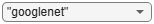This example shows how to classify an image using the pretrained deep convolutional neural network GoogLeNet.

GoogLeNet has been trained on over a million images and can classify images into 1000 object categories (such as keyboard, coffee mug, pencil, and many animals). The network has learned rich feature representations for a wide range of images. The network takes an image as input, and then outputs a label for the object in the image together with the probabilities for each of the object categories.

Load the pretrained GoogLeNet network. This step requires the Deep Learning Toolbox™ Model for GoogLeNet Network support package. If you do not have the required support packages installed, then the software provides a download link.

You can also choose to load a different pretrained network for image classification. To try a different pretrained network, open this example in MATLAB® and select a different network. For example, you can try `squeezenet`, a network that is even faster than `googlenet`. You can run this example with other pretrained networks. For a list of all available networks, see Load Pretrained Neural Networks.

`net =googlenet;`

The image that you want to classify must have the same size as the input size of the network. For GoogLeNet, the first element of the `Layers` property of the network is the image input layer. The network input size is the `InputSize` property of the image input layer.

`inputSize = net.Layers(1).InputSize`
```inputSize = 1×3 224 224 3 ```

The final element of the `Layers` property is the classification output layer. The `ClassNames` property of this layer contains the names of the classes learned by the network. View 10 random class names out of the total of 1000.

```classNames = net.Layers(end).ClassNames; numClasses = numel(classNames); disp(classNames(randperm(numClasses,10)))```
``` 'papillon' 'eggnog' 'jackfruit' 'castle' 'sleeping bag' 'redshank' 'Band Aid' 'wok' 'seat belt' 'orange' ```

Read and show the image that you want to classify.

```I = imread('peppers.png'); figure imshow(I)```Display the size of the image. The image is 384-by-512 pixels and has three color channels (RGB).

`size(I)`
```ans = 1×3 384 512 3 ```

Resize the image to the input size of the network by using `imresize`. This resizing slightly changes the aspect ratio of the image.

```I = imresize(I,inputSize(1:2)); figure imshow(I)```Depending on your application, you might want to resize the image in a different way. For example, you can crop the top left corner of the image by using `I(1:inputSize(1),1:inputSize(2),:)`. If you have Image Processing Toolbox™, then you can use the `imcrop` function.

### Classify Image

Classify the image and calculate the class probabilities using `classify`. The network correctly classifies the image as a bell pepper. A network for classification is trained to output a single label for each input image, even when the image contains multiple objects.

```[label,scores] = classify(net,I); label```
```label = categorical bell pepper ```

Display the image with the predicted label and the predicted probability of the image having that label.

```figure imshow(I) title(string(label) + ", " + num2str(100*scores(classNames == label),3) + "%");```### Display Top Predictions

Display the top five predicted labels and their associated probabilities as a histogram. Because the network classifies images into so many object categories, and many categories are similar, it is common to consider the top-five accuracy when evaluating networks. The network classifies the image as a bell pepper with a high probability.

```[~,idx] = sort(scores,'descend'); idx = idx(5:-1:1); classNamesTop = net.Layers(end).ClassNames(idx); scoresTop = scores(idx); figure barh(scoresTop) xlim([0 1]) title('Top 5 Predictions') xlabel('Probability') yticklabels(classNamesTop)```Szegedy, Christian, Wei Liu, Yangqing Jia, Pierre Sermanet, Scott Reed, Dragomir Anguelov, Dumitru Erhan, Vincent Vanhoucke, and Andrew Rabinovich. "Going deeper with convolutions." In Proceedings of the IEEE conference on computer vision and pattern recognition, pp. 1-9. 2015.